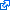# 1 paper accepted to CDC 21

Our paper on tight inner approximation of the positive-semidefinite cone  have been accepted to IEEE Conference on Decision and Control!T. Zheng, J. Guthrie, and E. Mallada, “Inner Approximations of the Positive-Semidefinite Cone via Grassmannian Packings,” in 60th IEEE Conference on Decision and Control (CDC), 2021, pp. 981-986.

We investigate the problem of finding tight inner approximations of large dimensional positive semidefinite (PSD) cones. To solve this problem, we develop a novel decomposition framework of the PSD cone by means of conical combinations of smaller dimensional sub-cones. We show that many inner approximation techniques could be summarized within this framework, including the set of (scaled) diagonally dominant matrices, Factor-width k matrices, and Chordal Sparse matrices. Furthermore, we provide a more flexible family of inner approximations of the PSD cone, where we aim to arrange the sub-cones so that they are maximally separated from each other. In doing so, these approximations tend to occupy large fractions of the volume of the PSD cone. The proposed approach is connected to a classical packing problem in Riemannian Geometry. Precisely, we show that the problem of finding maximally distant sub-cones in an ambient PSD cone is equivalent to the problem of packing sub-spaces in a Grassmannian Manifold. We further leverage the existing computational methods for constructing packings in Grassmannian manifolds to build tighter approximations of the PSD cone. Numerical experiments show how the proposed framework can balance accuracy and computational complexity, to efficiently solve positive-semidefinite programs.

``````@inproceedings{zgm2021cdc,
abstract = {We investigate the problem of finding tight inner approximations of large dimensional positive semidefinite (PSD) cones. To solve this problem, we develop a novel decomposition framework of the PSD cone by means of conical combinations of smaller dimensional sub-cones. We show that many inner approximation techniques could be summarized within this framework, including the set of (scaled) diagonally dominant matrices, Factor-width k matrices, and Chordal Sparse matrices. Furthermore, we provide a more flexible family of inner approximations of the PSD cone, where we aim to arrange the sub-cones so that they are maximally separated from each other. In doing so, these approximations tend to occupy large fractions of the volume of the PSD cone. The proposed approach is connected to a classical packing problem in Riemannian Geometry. Precisely, we show that the problem of finding maximally distant sub-cones in an ambient PSD cone is equivalent to the problem of packing sub-spaces in a Grassmannian Manifold. We further leverage the existing computational methods for constructing packings in Grassmannian manifolds to build tighter approximations of the PSD cone. Numerical experiments show how the proposed framework can balance accuracy and computational complexity, to efficiently solve positive-semidefinite programs.},
author = {Zheng, Tianqi and Guthrie, James and Mallada, Enrique},
booktitle = {60th IEEE Conference on Decision and Control (CDC)},
doi = {10.1109/CDC45484.2021.9682923},
grants = {EPCN-1711188, CAREER-1752362, AMPS-1736448, TRIPODS-1934979},
month = {12},
pages = {981-986},
record = {presented Dec. 2022, accepted Jul. 2021, submitted Mar. 2021},
title = {Inner Approximations of the Positive-Semidefinite Cone via Grassmannian Packings},